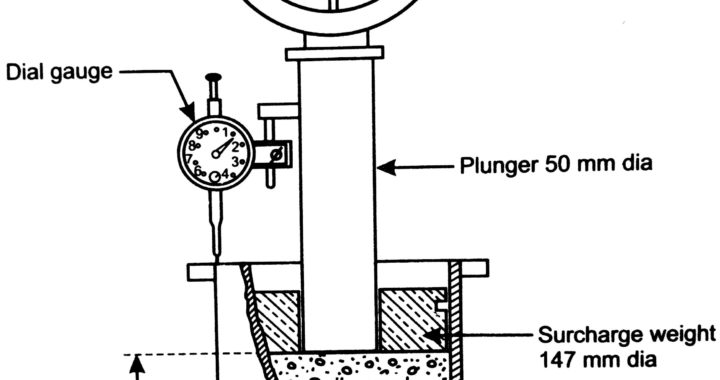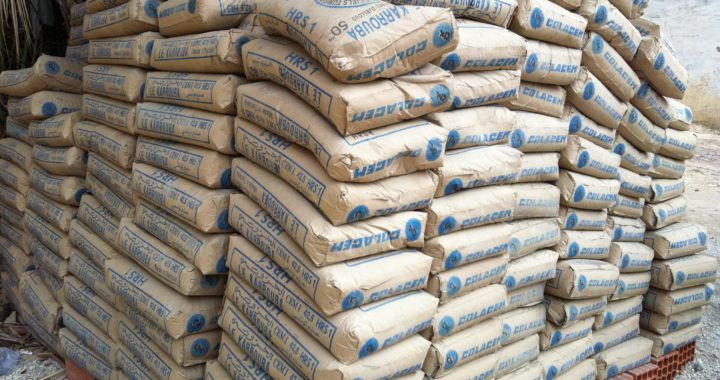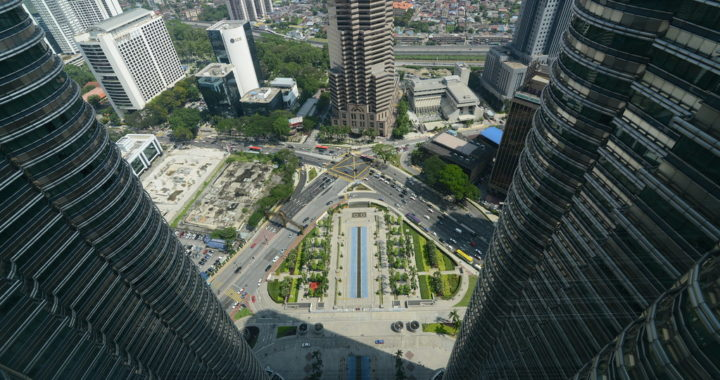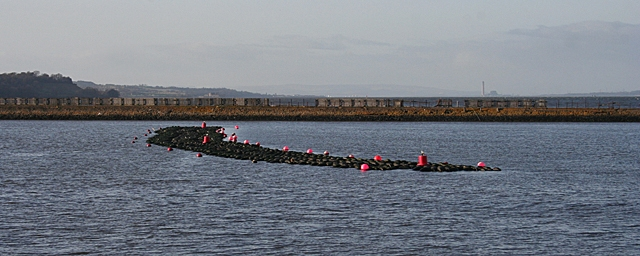Fri. Nov 22nd, 2019

# Design parameters of Embankment with revetment – River training

##### Design parameters of Embankment with revetment – River training

This article is about river training works and design parameters of river training components such as the design of Embankment with revetment, Spur, stud Groynes, Calculation of discharge, calculation of spacing between the embankment, channelization, etc.

### Embankment

• The flood return period 25 years and free board as per BIS (1.5m).
• The alignment of embankment should be fixed 1.5  times of Lacey’s width from central line of the river. But in practice, this may not possible near the village and beyond ch 30+00
• Design thickness of 0.2 m for pitching in gabion for slope protection.
• Countryside, side-slope =2:1 and riverside, side slope = 2:1 for the embankment, for height up to 5 m and top width =5m, bottomland width up to 28m including a plantation.
• Embankment will be widened in every 1.00 km for vehicle crossing in 50m length and 10 m width.
• 50 cm sweet soil covers on both side and top of Embankment.

### Spur

• The spur shall be designed for 50 years return period at designed discharge  “Q “ cumecs.
• Freeboard shall be as per BIS specification 1.5m and IS practice Top of the spur shall be the minimum 4m for earth fill spur.
• Crated boulder pitching  0.5m thick in machine-made crate single layers (0.5 m thick layer ) shall be provided in the semicircular nose only of slope,  transition, from nose to shank and for another 25m of the shank in U/S face of the spur and D/S face, 0.3m thick crated boulder pitching shall be provided , transition, from nose to shank and next  25m length of the shank.
• Size of the apron in nose 12m x 0.4m and it shall be 10 gauge HZC hand made crate of mesh 10cm x 10cm GI Crate. The priority of Machine-made Hexagonal of 10×12 mesh type / or mesh 6cm x 8cm mesh reno mattress for slope should be given, the life of machine-made crate is much high and effective.
• For transition, from nose to shank and 30m length in the D/S face of the spur and launching apron shall be of 6m x 0.4m
• Apron shall be laid at a low water level (LWL)
• For smooth flow, nose should be semi-circular.
• U/S and D/S of spur at bank connection should be reverted with embankment in 15 m lengths (launching and slope pitching ) both side
• River  bed material should be filled up to the lowest water level (LWL) for construction of spur for the laying of crates in deep water

### Revetment

• Thickness of slope pitching will be of 0.2 m in machine-made Reno mattress gabion over Geo-Textile in slope and for launching hand mad crates from HZC wire crates of 0.4m thick mess size 10cm x 10 cm and width
• All revetment works shall be up to design flood level.
• End anchorage shall be provided in the revetment.
• Apron  shall be laid at LWT (lowest water level), this is very important

### Water way fixation

Lacey’s waterway is 4.75 (Q)^1/2

• It is accepted to provide the width twice the lacey’s width confined bank with revetment.

### Thickness of launching Apron

The thickness of the launching apron is 0.4 to 0.7 m.

### Length and spacing of Spur

The placement of Spur/Stud in general is governed by the purpose and configuration of the river. In most of the river training works, the spacing of the spurs have been noticed to be 2 to 3 times studs length. The series placements of spurs/studs along the banks of the River should have effectively protected the intended purpose wherever anti-erosion measures needs. This varies from year to year and location of spur can be shifted or added wherever erosion occurs.

The length of the spur is dependent on the  width of the river channel and the extent of protection work needed . In general, the spur length provided should not exceed 1/10 of the width of the river.

## Spacing of Embankment

In general, embankments are aligned at a minimum spacing of three times Lacy’s waterway.

If the design discharge of the River is adopted Q  cumecs. For embankment construction, Lacy’s waterway width comes to be  4.75 X Q^(1/2) m.  For spur construction in the main channel, the total width is about a minimum of  300m to 500m.

Embankment should be aligned not less than 3P (where, p= perimeter ) except the thickly populated village on both the is mor banks. The area where river width is more than 3P, bench terracing from bamboo piles and plantation should be adopted.

Design of spur and Revetment

• Design Discharge

The high flood Design discharge would be calculated by different methods.

For example(one of the River in India, Bihar) JICA master plan study report shows  Q 50 = 1150 cumecs near Down-stream(D/S) end including most of the tributaries.

According to Flood frequency analysis and discharge observed is approximately 1000 cumecs.

Followings  methods are also used to calculate the discharge and maximum is taken.

a. Water and Energy Commission Secretariat Approach (WECS)

The 2-year and 100 year return period instantaneous floods (Q2 and Q100 respectively) are calculated.

 Q2          = 1.8767 x (area below 3000 m) 0.8783 Q100       = 14.630 x (area below 3000 m) 0.7343 The flood flows for any other return period R is then calculated by: QR    = e (InQ2 + S x α) Where, S is the standard deviation of natural logarithm of annual floods and α stands for a standardized normal variant for particular period R. The values α for different return period is given as below Return Period, years 2 5 10 20 50 100 200 Value of α 0 0.842 1.282 1.645 2.054 2.326 2.576 S can be calculated as follows: S = (In (Q100/Q2))/2.326 In = LOG(number,(2.71828))

b. ModifiedDickens’s Method

 This is one of the methods widely used in Nepal and north India for the estimation of peak discharge. The peak discharge can be calculated by using the following formula: QT  = CT x A3/4 Where CT is for the return period T, given by: CT  = 2.342 Log (0.6T) Log (1185/P) + 4 Where, P = 100(AS + 6)/A AS  = snow-covered area of the total catchment area.

#### ·         Depth of Flow Calculation

 Lacey’s waterway = L = 4.75*Q^(1/2) = 161.08 m Adopted Waterway as per site condition= 250 m

The cross section of the spot is assumed to be rectangular shape taking consideration of the shape of existing river section.

Water depth = d

Wetted Area = A=B*d

Wetted Perimeter = p = B+2d

Hydraulic Radius = H = A/p =( B*2d)/(B+2d)

Velocity = V = 1/n*H^2/3*S^1/2

Discharge = Q = V*A

#### ·         Design of Embankment & Protection

Design Data

Design Discharge                              Q50 =    1150 Cumecs

Water depth                                      d50 =     1.81        m

Adopt   d50 =     2.00        m from bed of River

Average velocity              u =          2.53        m/s

1. Embankment Design
 Free board Fb = 1.5 m Side slope on both sides 1:2 S = 2 Assumed seepage line slope  1:4 Ss = 4 Top width T = 5 m Height of the Embankment above River bed hemb = 3.5 m Height of the Embankment above River bank hemb = 2 m Waterways required 161.08 m Provided waterways 250 m Required Bottom width  from seepage consideration = 12 m Provided Bottom width of the embankment 13 m
• Bank Revetment Design

Thickness of the Revetment is given by

 Where, Slope stability factor φc = 0.75 for stone pitching Turbulence factor KT = 1.5 for turbulent and non-uniform flow Mean equivalent roughness height Kr = 0.95 d =   1.43 m Depth and velocity distribution factor Kh From calculation Kh = 0.45
 Relative density Δm = (ys-yf)(1-η)/yf Where, Density of pitching material ys= 2,000 Kg/m3 Density of water yf= 1,000 Kg/m3 Porosity of boulder filled boxes η= 0.35 From calculation Δm = 0.65
 Slope reduction factor Ks = (1-Sin2α/Sin2Ø)1/2 where α = slope angle Ø = angle of repose In present case α = 26.565 ° Ø = 40 Results Ks = 0.718 Dimensionless Critical shear stress ϴc = 0.06 Required  revetment thickness Dn = 0.210 m Provided revetment thickness 0.30 m OK

### ·         Launching Apron Design

 Lacey’s normal scour depth below water surface R = 0.47 (Q/f)1/3 f = silt factor =1.76 (dmm)1/2 dmm = 1 f = 1.76 Calculated R = 4.078 Take multiplying factor x = 1.25 Depth of Scour hole below river bed D = 3.1 m Assuming the apron launches at 1:1.50 (V:H) slope The required width of launching apron = = 1.80*D = 5.58 m Provided launching apron width 6.00 m Required apron thickness for gabion crated boulder ~1.50*revetment thickness = 0.32 Provided Launching apron thickness 0.40 m

### ·         Stud Design

 Lacey’s normal scour depth  R= 0.47 (Q/f)1/3 where, Q = Discharge m 3/s f = Silt factor = 1.76 (dmm)1/2 adopted dmm = 1 f = 1.760 Q= 1150.00 R= 4.08 Depth of water above Effective Length of stud (Leff)  < (15% of the Waterway) = 24.16 m Total length of stud provided = 12.00 m Effective length of stud with 5m idle length 7.00 m For 35% permeability and 90° orientationϴ= 17.5° Spacing of studs S = LCot ϴ = 44.78 Take multiplying factor x = 1.25 Depth of Scour hole below river bed D = 4.31 m Assuming the apron launches at 1:1.5 (v:h) slope The required width of launching apron = = 1.80*D = 7.758 m Provided Parameters Length of Stud Ls= 12 m Spacing of studs S= 40 m Length of apron La= 9 m

##### Conclusion

In this way we can design and fix the Design parameters of river training woks. One of the additional parameter for design of river training work is bio-Engineering works. For the tips on the bio-engineering works read the other post “River training and bio-engineering”.

(Visited 191 times, 1 visits today)

## 3 thoughts on “Design parameters of Embankment with revetment – River training”

#### You may have missed#### California Bearing Ratio(CBR Test) of Subgrade Soil -Procedure, apparatus, and use for pavement Design#### Types of cement their properties and Uses#### High strength concrete properties, strength, admixture, and mix design